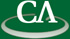## Sparse Polynomial Interpolation and the Fast Euclidean Algorithm.

### Soo Go, CECM, Simon Fraser University

June 20th, 2012 at 3:00pm in K9509.

```We introduce an algorithm to interpolate sparse multivariate polynomials
with integer co- efficients. Our algorithm modifies Ben-Or and Tiwari’s
deterministic algorithm for interpolating over rings of characteristic
zero to work modulo p, a smooth prime of our choice. We present
benchmarks comparing our algorithm to Zippel’s probabilistic sparse
interpolation algorithm, demonstrating that our algorithm makes fewer
probes for sparse polynomials.

Our interpolation algorithm requires finding roots of a polynomial in
GF(p)[x], which in turn requires an efficient polynomial GCD algorithm.
Motivated by this observation, we review the Fast Extended Euclidean
algorithm for univariate polynomials, which recursively computes the GCD
using a divide-and-conquer approach. We present benchmarks for our
implementation of the classical and fast versions of the Euclidean
algorithm demonstrating a good speed-up. We discuss computing resultants
as an application of the fast GCD algorithm.
```